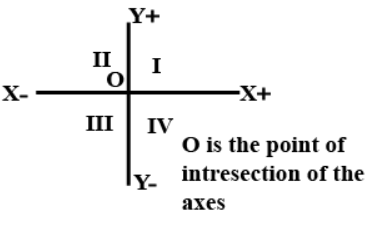QuestionAnswersVerified
25.1K+ Views
Hint: First, start by defining what a quadrant and abscissa is. Draw a diagram which shows all the different quadrants on the coordinate axes. This diagram will help to identify the quadrants where the abscissa and the ordinate are positive or negative. From the diagram and the definitions, find the sign in each of the quadrants and find the final answer.

Complete step-by-step solution -
In this question, we need to find the quadrants in which the abscissa of a point is positive.
Let us start by defining what a quadrant actually is.
The axes of a two-dimensional Cartesian system divide the plane into four infinite regions, called quadrants, each bounded by two half axes. These are often numbered from 1st to 4th and denoted by Roman numerals.
Following is the diagram which shows the 4 quadrants.Now, let us define what abscissa actually is.
The abscissa is the x coordinate of a point on the coordinate plane. The distance along the horizontal axis.
A coordinate is an ordered pair of numbers in which the first number is the abscissa i.e. the measurement along the X axis.
Using all this information, we can conclude the following:
The signs of the coordinates in:
The first quadrant is (+, +)
The second quadrant is (−, +)
The third quadrant is (−, −)
The fourth quadrant is (+, −)
So, the abscissa of the point is positive in the first and fourth quadrant.
Hence, option (d) is correct

Note: In this question, it is very important to know what a quadrant is and what abscissa is. The abscissa is the x coordinate of a point on the coordinate plane. The distance along the horizontal axis. Using this information, find the signs in each of the quadrants.